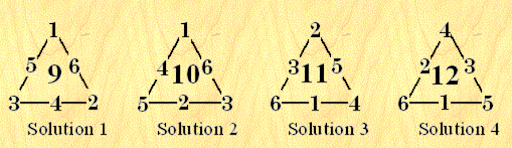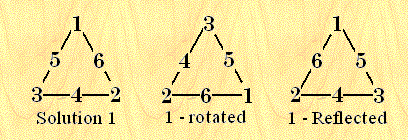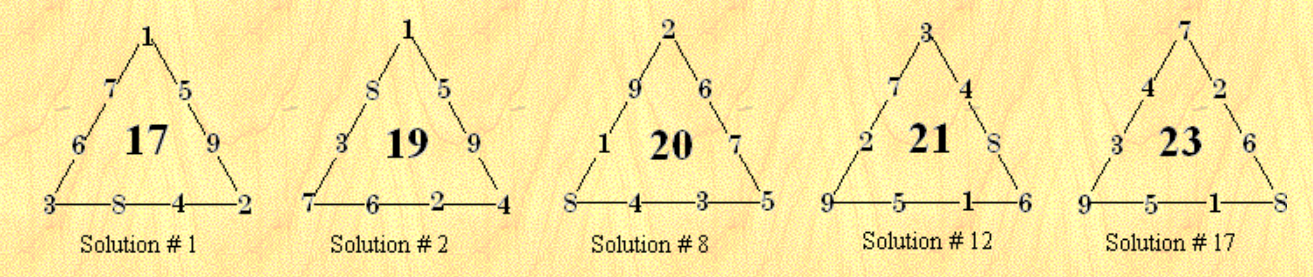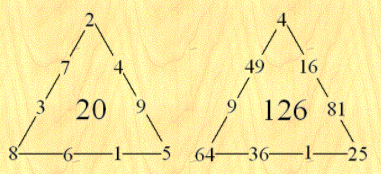Math Puzzles
Do you want to know the secrets of the Magic Triangle?
19.5k views

 1. Introduction 2. History of magic triangles 3. Order 3 Perimeter Magic Triangles 4. Order 4 Perimeter Magic Triangles 5. Conclusion 6. About Cuemath 7. FAQ's 8. External References

November 23 2020

## Introduction

### What do you know about the Magic triangle?

A magic triangle is an arrangement of integers on the sides of a triangle, each side having the same number of integers.

### Simple example:

1. Use each of the numbers from 1 to 9.
2. Write one number in every circle.
3. The numbers on each side of the triangle must add up to 17.

## History of magic triangles

In 1972, Terrel Trotter, Jr., then a math teacher in Urbana Illinois, published an article called Normal Magic Triangles of Order n . In 1974, he published a follow-up article called Perimeter-magic Polygons . In it he introduced the term Perimeter-magic Polygon and generalized it to include other polygonal shapes. Since that time, other writers have expanded on the same theme.

## Order 3 Perimeter Magic Triangles

Order-3 is the simplest perimeter- magic triangle, except for order 1 which is trivial (there are no order 2 perimeter-magic objects). Unlike magic squares, cubes, etc., there are multiple magic constants for the same order.Here I show the only PMPs of order 3.

Solution 4 is the compliment of solution 1, and solution 3 is the complement of solution 2 (see below).

### Complements

For any given solution, there is always a complement solution. It is obtained by subtracting each number in turn from the sum of the first and last numbers in the series. So if your solution uses the numbers from 1 to 9, another solution is obtained by subtracting each number from 10 (ie. The sum of the first and last numbers in the series). This feature applies to magic squares, cubes etc. as well.

The new solution obtained in this way must then be normalized by rotation and/or reflection, to comply with the above

For higher orders, with many solutions, it is necessary to only find solutions for the smallest half of the possible sums. The higher magic sums may then be obtained simply by complementing these lower solutions.

### Rotations and ReflectionsRotations and Reflections are not considered unique solutions. Each basic solution has two rotations and each of these has one (horizontal) reflection, for a total of 6 apparently different solutions. For other polygons, these numbers would obviously vary.

## Order 4 Perimeter Magic Triangles

### Problem

There are 18 basic solutions for order 4 PM Triangles. Note that there are no solutions with magic sums of 18 and 22.

The number of solutions for each sum should be symmetrical.

Sum 17 19 20 21 23
# of solution 2 4 6 4 2=18

The list below contains all solutions for order 4 PM Triangles.

S=Side sum (i.e. the magic constant, V=Vertex sum, C=the complement solution #, CS=the complement side sum, CV=the complement vertex sum. Columns A, B, and C are the vertices

 # A B C S V C 1. 1 5 9 2 4 8 3 6 7 17 6 17 2. 1 5 9 4 2 6 7 3 8 19 12 12 3. 1 6 8 2 5 7 3 4 9 17 6 18 4. 1 6 8 4 3 5 7 2 9 19 12 14 5. 1 6 8 5 2 4 9 7 3 20 15 6 6. 2 4 9 5 1 6 8 3 7 20 15 5 7. 2 5 9 3 1 8 7 4 6 19 12 10 8. 2 6 7 5 3 4 8 1 9 20 15 8 9. 2 6 8 3 4 5 7 1 9 19 12 13 10. 3 2 9 7 1 5 8 4 6 21 18 7 11. 3 4 8 5 2 6 7 1 9 20 15 11 12. 3 4 8 6 1 5 9 2 7 21 18 2 13. 3 5 6 7 2 4 8 1 9 21 18 9 14. 3 5 7 6 2 4 9 1 8 21 18 4 15. 4 2 9 5 1 8 6 3 7 20 15 15 16. 4 3 8 5 2 7 6 1 9 20 15 16 17. 7 2 6 8 1 5 9 3 4 23 24 1 18. 7 3 5 8 2 4 9 1 6 23 24 3

### Some example diagramsSolution # 17 is the normalized complement of solution #1, and # 12 is the normalized complement of #2. Number 8 is it’s own complement.Solution number 6 is of special interest because it is magic. It was originally discovered by David Collison of California, USA . When each number is squared, the triangle is still perimeter magic. This time with a sum of 126.

## Conclusion

Math should be fun and exciting for children and it is not possible to make it so without the help of creative ways to teach maths. Keeping this in mind, we have designed a fun and engaging math puzzle 'riddle me this' that will help your child understand math in a fun way.

Math puzzles enable children to visualize concepts and thus understand math concepts better and faster. Through better visualization, they retain the concepts for a longer time in their mind. Math puzzles also improve their problem-solving skills and mathematical thinking abilities which is very important in the real world.

Magic Triangles are really a series of problems that grow gradually harder. These are a perfect way to practice one-digit addition in a context that promotes logical thinking and problem-solving.

Instructions to solve Magic Triangle?

Instructions: Arrange the numbers for each triangle (1-6 for the 3 x 3 x 3 triangle; 1-9 for the 4 x 4 x 4 triangle) so that the sum of numbers on each side is equal to the sum of numbers on every other side. For the small triangle, arrange the numbers so that the sum of each side equals 9.

Cuemath, a student-friendly mathematics platform, conducts regular Online Classes for academics and skill-development and their Mental Math App, on both iOS and Android, is a one-stop solution for kids to develop multiple skills. Understand the Cuemath Fee structure and sign up for a free trial.